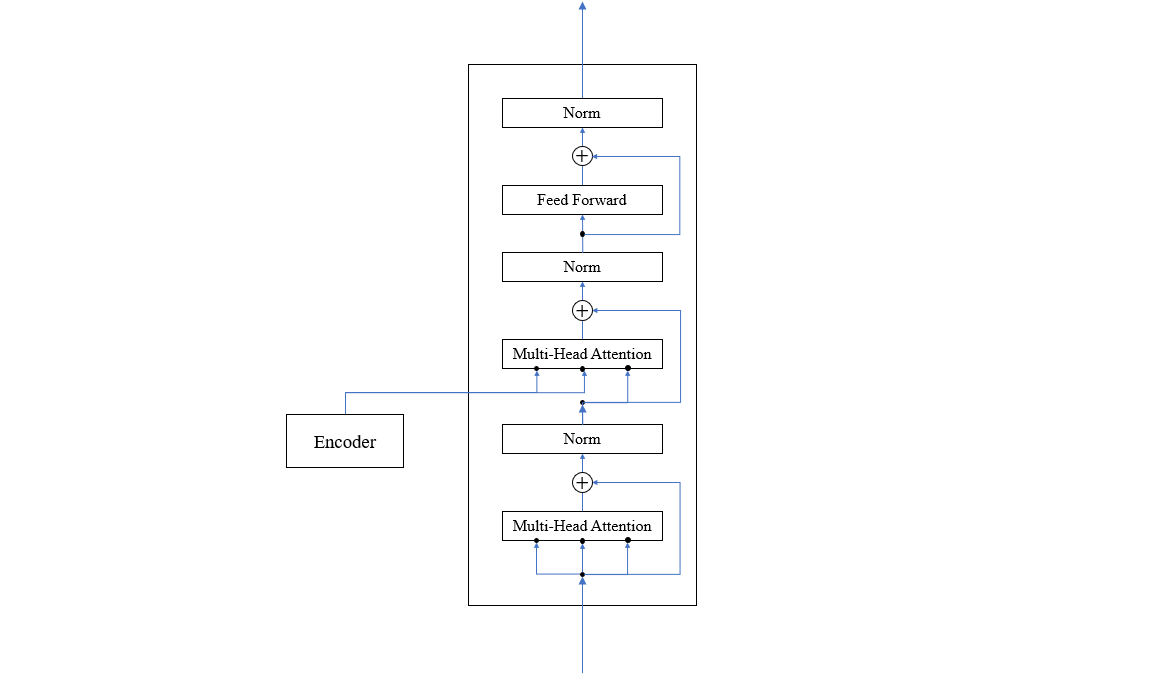Fenrier Lab

# 关于 Transformer 架构的详细分析

##### 自注意力机制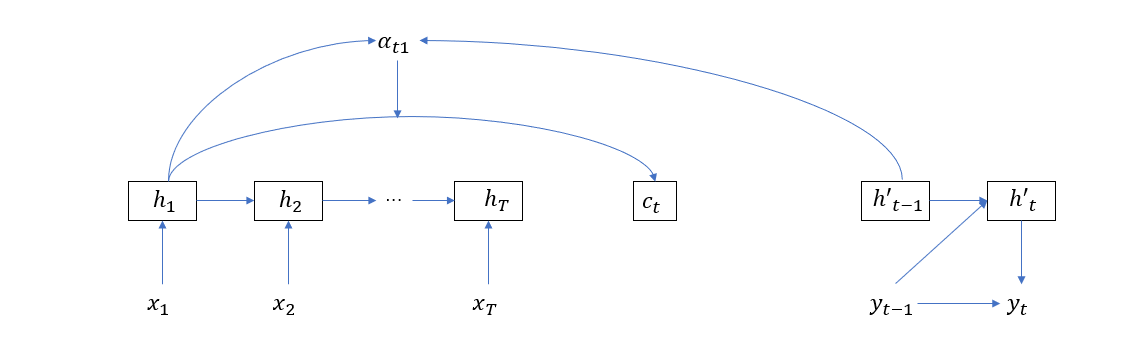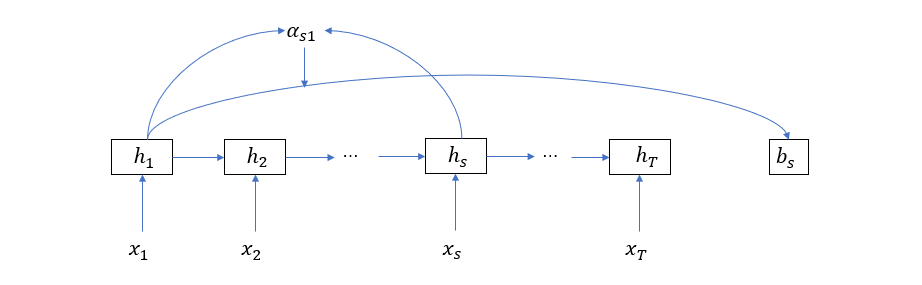\begin{aligned} b_s &= \sum_{i=1}^T \alpha_{si} h_i = H \alpha_s \\ \alpha_{j} &= softmax(e_j)\\ e_{ji} &= a(h_j, h_i) \end{aligned}

e_j = \left[ \begin{aligned} e_{j1}\\e_{j2}\\\vdots\\e_{jT} \end{aligned} \right] = \left[ \begin{aligned} h_1^\top h_j\\h_2^\top h_j\\\vdots\\ h_T^\top h_j \end{aligned} \right] = \left[ \begin{aligned} h_1^\top\\h_2^\top\\\vdots\\ h_T^\top \end{aligned} \right]h_j = H^\top h_j

\begin{aligned} b &= [b_1 \quad b_2 \quad ... \quad b_T] \\ &= H [\alpha_1 \quad \alpha_2 \quad ... \quad \alpha_T]\\ &= H softmax([e_1 \quad e_2 \quad ... \quad e_T] )\\ &= H softmax( H^\top[h_1 \quad h_2 \quad ... \quad h_T])\\ &= H softmax(H^\top H) \end{aligned}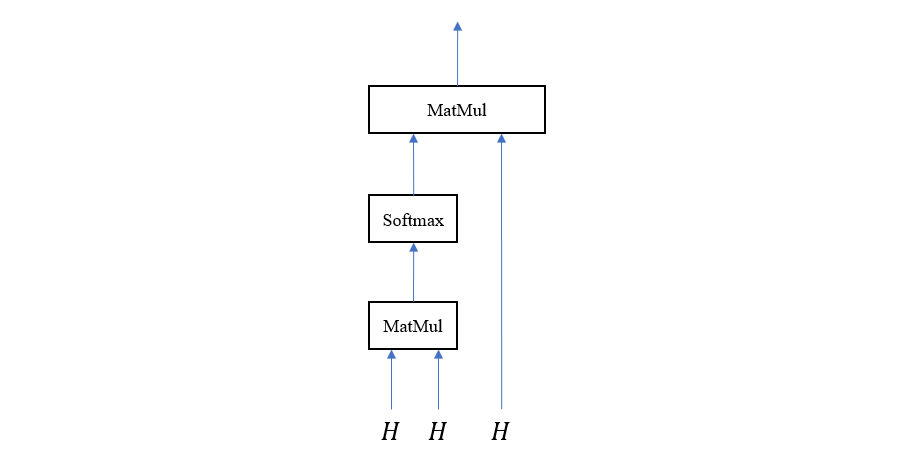##### 关于 Query, Key 和 Value 的解释

c_t =\sum_{i=1}^T \alpha_{ti} h_i = \alpha_{t1} \left[\begin{aligned} h_{11} \\h_{12}\\... \\h_{1n} \end{aligned}\right] + \alpha_{t2} \left[\begin{aligned} h_{21} \\h_{22}\\... \\h_{2n} \end{aligned}\right] +...+ \alpha_{tT} \left[\begin{aligned} h_{T1} \\h_{T2}\\... \\h_{Tn} \end{aligned}\right] = \left[ \begin{aligned} \sum_{i = 1}^T \alpha_{ti} h_{i1}\\\sum_{i = 1}^T \alpha_{ti} h_{i2}\\...\\\sum_{i = 1}^T \alpha_{ti} h_{in} \end{aligned} \right]

$c_{tk} = \sum_{i=1}^T \alpha_{ti}h_{ik}$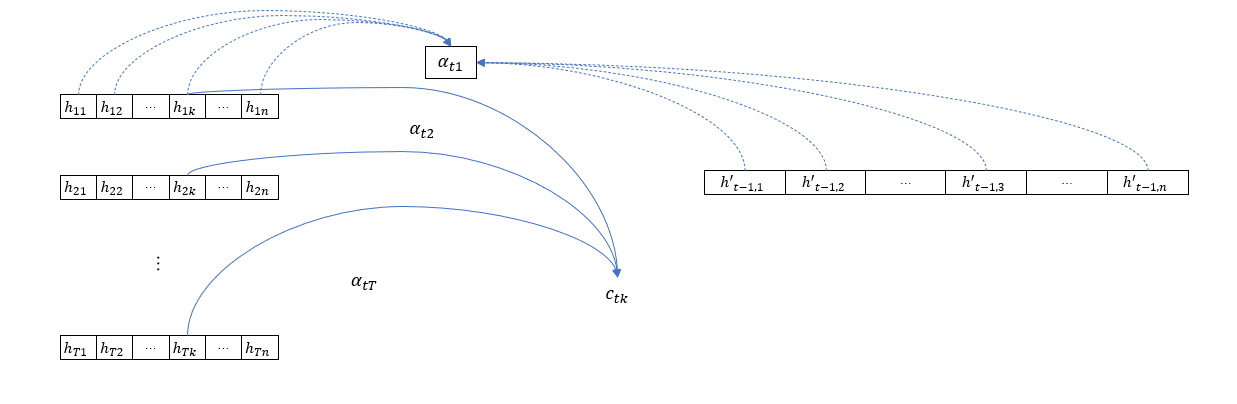\begin{aligned} c_{tk} &= v_k\alpha_{t}\\ &=v_k [\alpha_{t1} \quad \alpha_{t2} \quad ...\quad \alpha_{tT}]^\top\\ &=v_k softmax({h'}_{t-1}^\top [h_1\quad h_2 \quad ... \quad h_{T}])^\top\\ &=v_k softmax(H^\top h'_{t-1}) \end{aligned}

\begin{aligned} K:&\quad V\\ h_1: &\quad h_{1k}\\ h_2: &\quad h_{2k}\\ ...\\ h_T: &\quad h_{Tk} \end{aligned}

$c = V softmax(K Q)$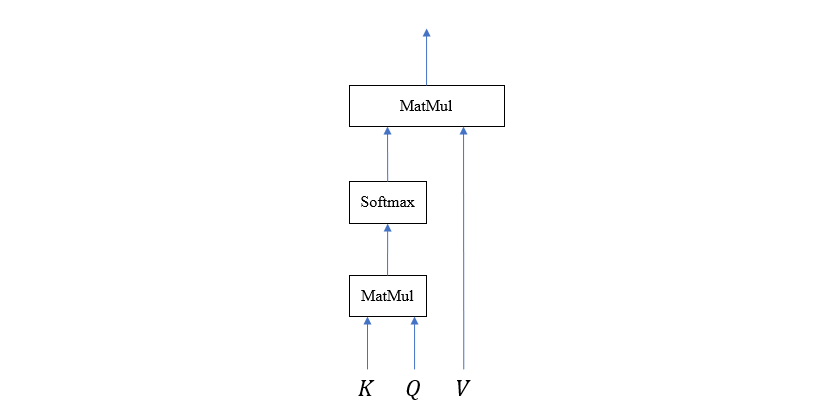##### Transfomer 的自注意力模块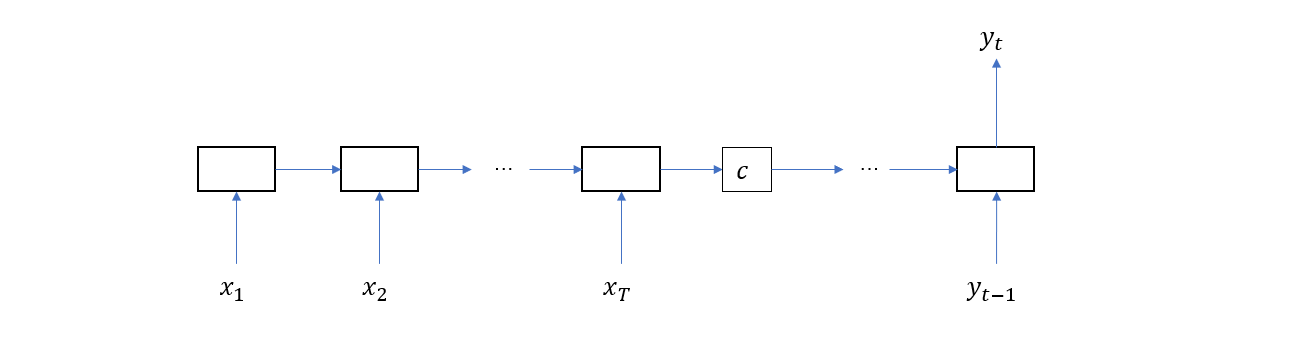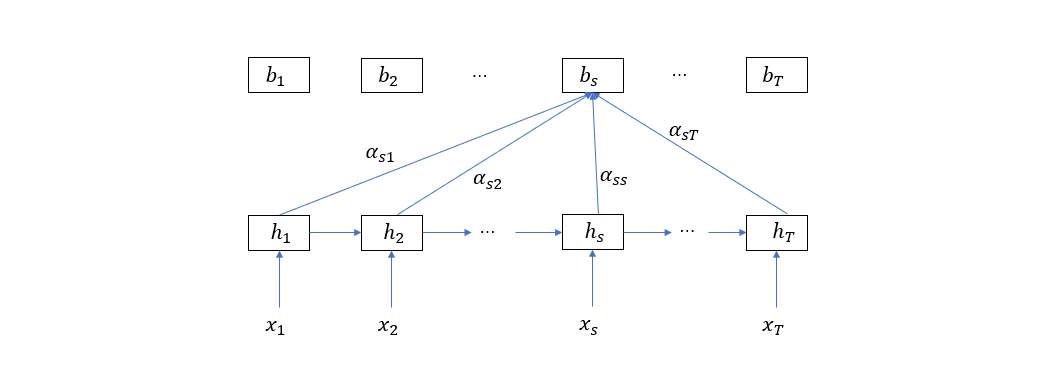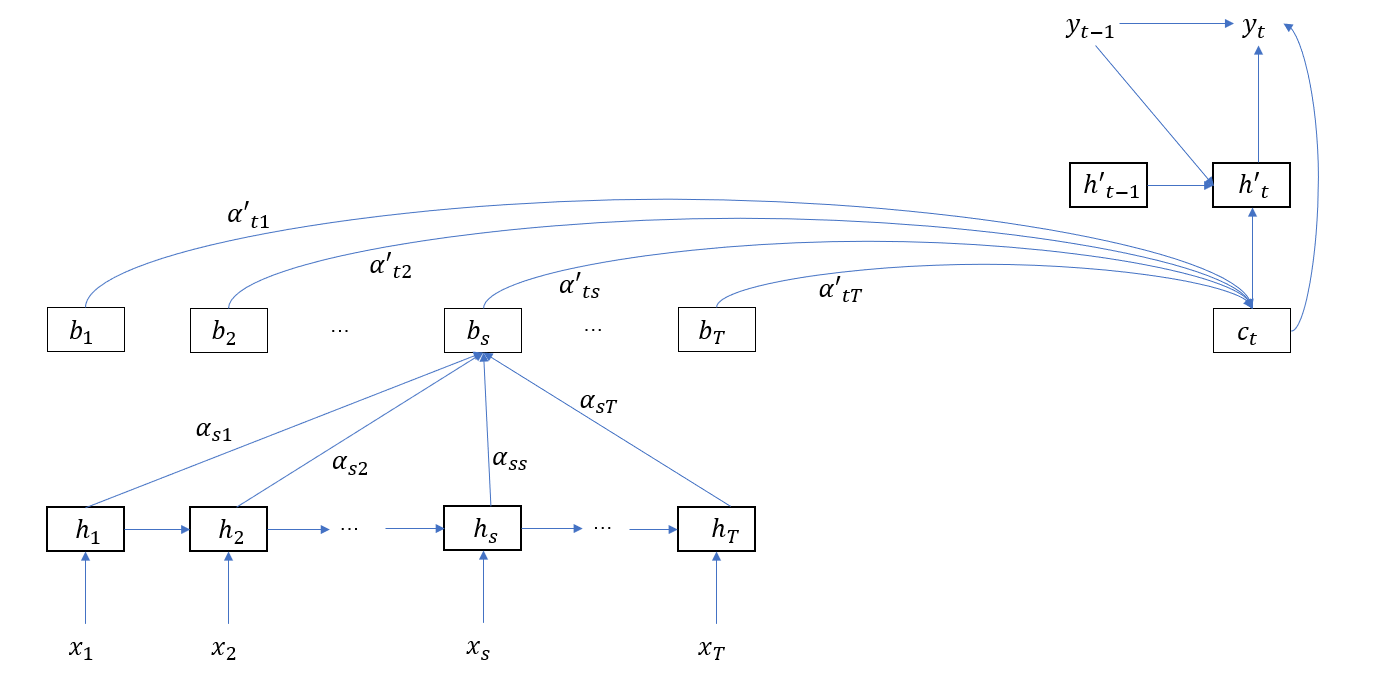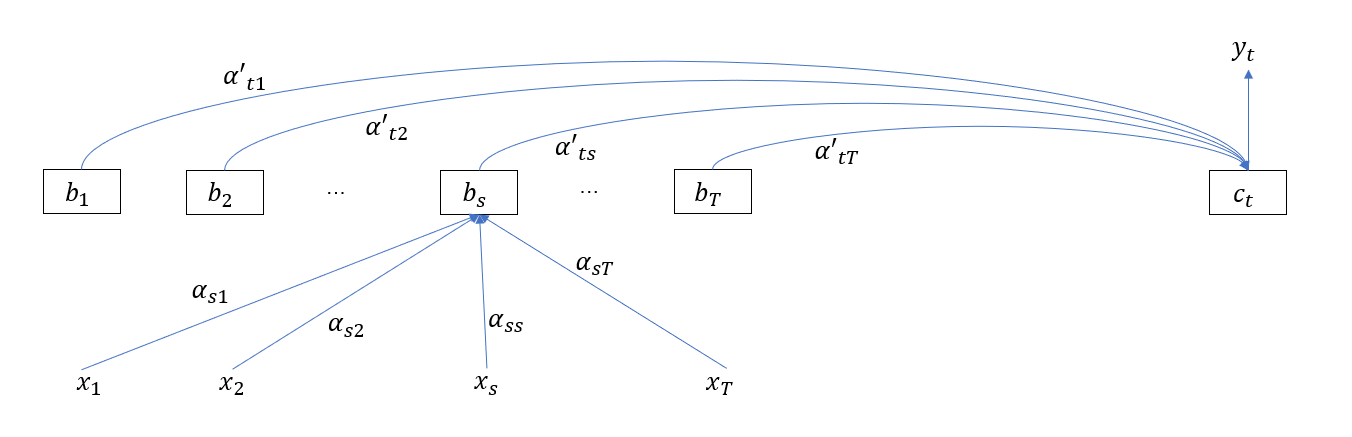$b_s = \sum_{i = 1}^T \alpha_{si} x_i$

$b = [b_1 \quad b_2 \quad ... \quad b_T] = X softmax(X^\top X)$

$b = V softmax(K Q)$

Multi-Head 注意力是以上一节介绍的点积注意力模块为基本块而组成的，它的目的是构造多个子空间的注意力向量。所以，首先需要将输入向量映射到子空间，方法是使用全连接层映射，具体表现在乘以一个矩阵 $$X_s = X W$$。假设输入向量的维度为 $$d=512$$，需要构造 $$h=8$$ 个子空间注意力，则每个子空间的维度为 $$64$$，于是矩阵 $$W$$ 的尺寸就为 $$512 \times 64$$，子空间维度为 $$64$$，将这 8 个子空间注意力向量拼接起来又得到一个 512 维的联合注意力向量。这样一来，Multi-Head 注意力的输入和输出便和普通注意力模块的输入输出统一起来了，前者可以无缝替换后者。计算图如下所示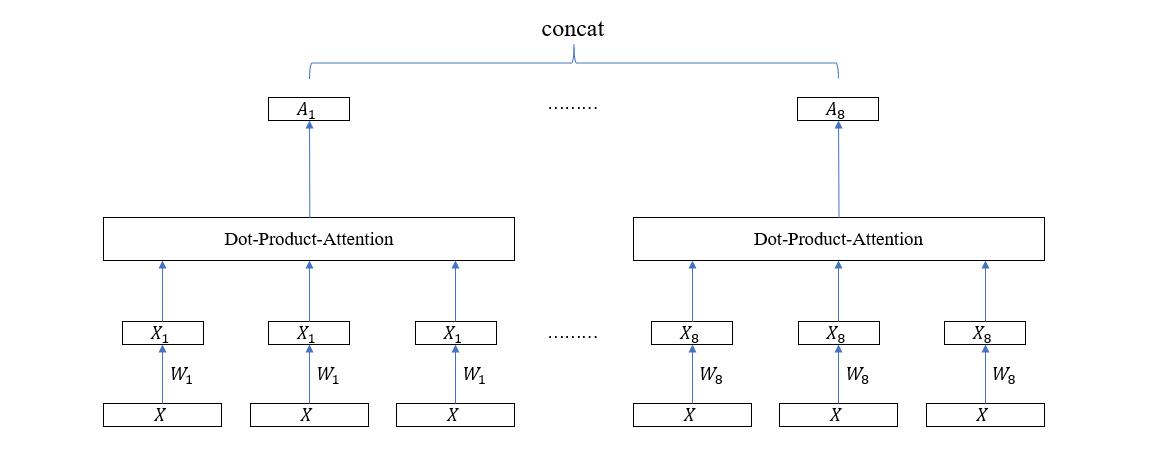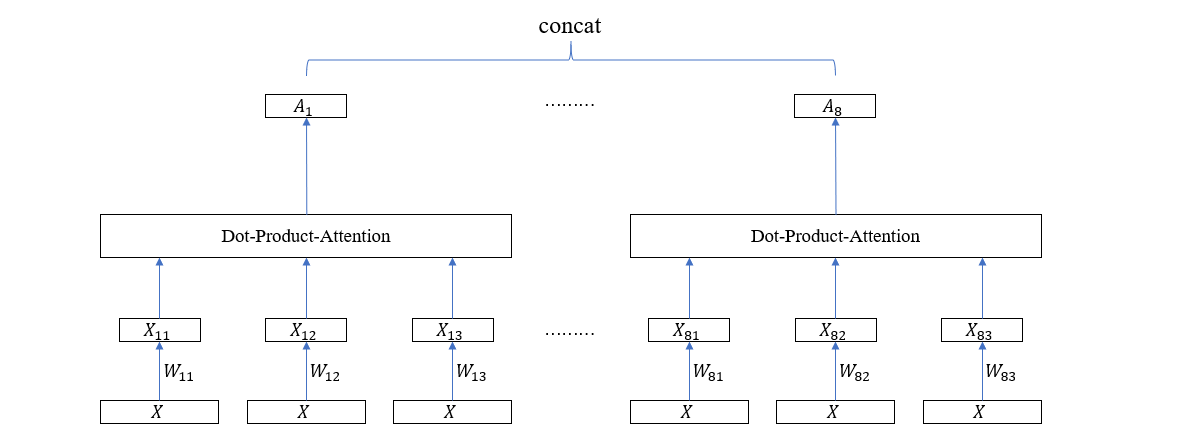##### Transformer 的 Encoder-Decoder 结构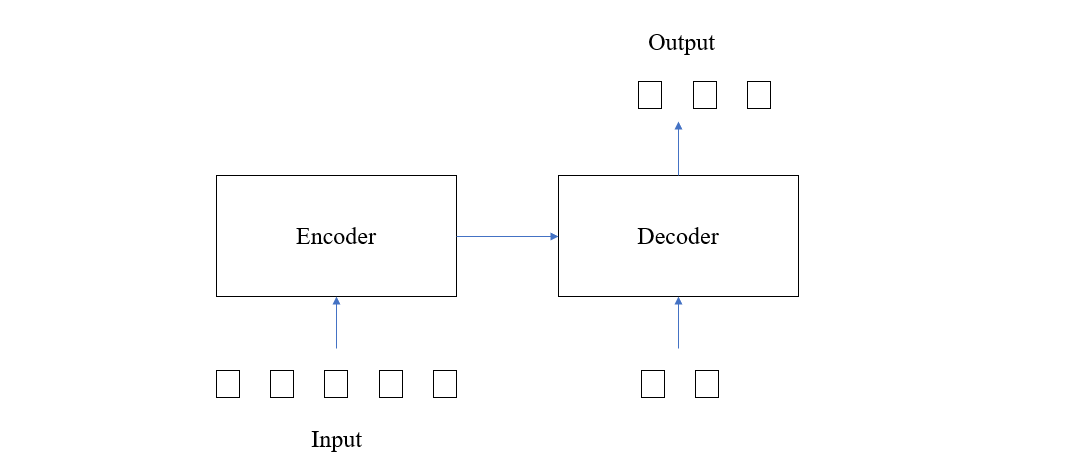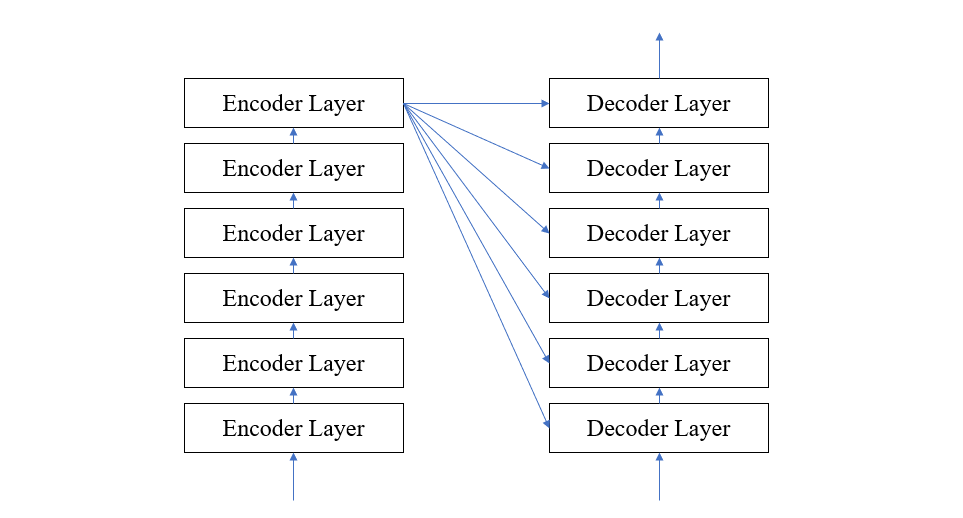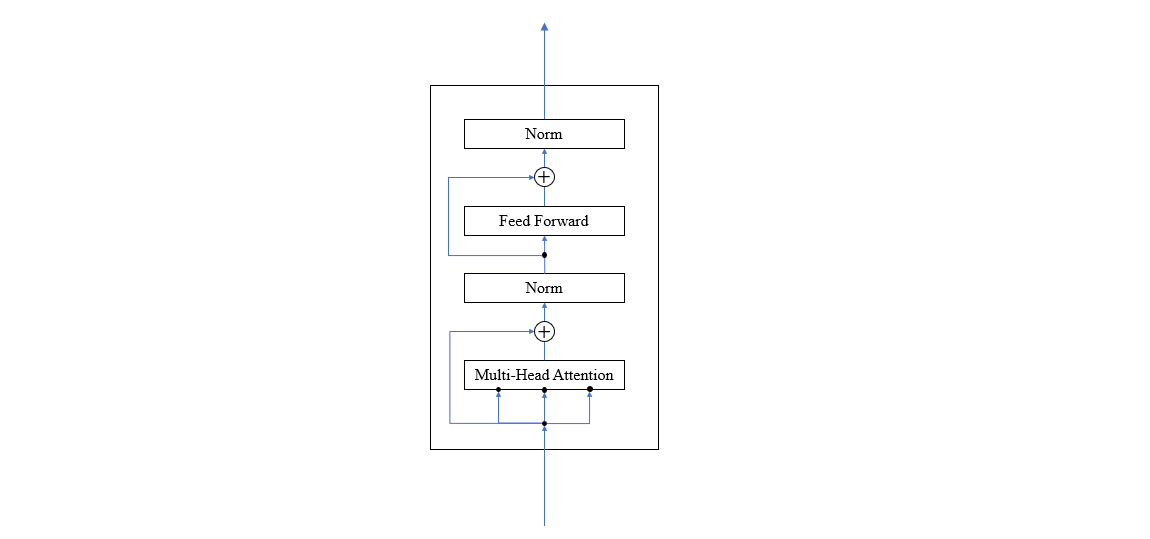Decoder Layer 的情况要复杂一点，它一方面要接收 Encoder 给的中间表示 $$E$$，另一方面要接收上一轮的预测序列 $$P_{t-1}$$。在 Decoder Layer 中，首先将 $$P_{t-1}$$ 使用注意力模块进行编码得到 Query 矩阵 $$Q$$，然后令 $$E^\top$$ 作为 key 矩阵 $$K$$，$$E$$ 作为 value 矩阵 $$V$$，把它们输入注意力模块计算跨结构的注意力，最后输入全连接层，如下图所示